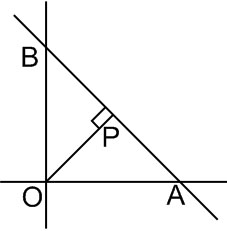# Locus of mid point of the portion between the axes of   is constant is Option 1) Option 2) Option 3) Option 4)

As we learnt in

Normal form -

- wherein

p is the length of perpendicular segment from origin and  is the angle made by this perpendicular with +ve -axis.Point A is:

Point B is:

We have,

Option 1)

This option is incorrect

Option 2)

This option is incorrect

Option 3)

This option is incorrect

Option 4)

This option is correct

Exams
Articles
Questions